# HSPT Math : How to add and subtract decimals

## Example Questions

← Previous 1

Jenny is getting ready for school. She bought school supplies on a tax-free day. She bought notebooks for $1.25 each and crayons for$0.90 each. Jenny bought two more notebooks than crayons. She paid with a ten dollar bill and got $1.05 in change. How many notebooks did she buy? Possible Answers: 2 4 5 1 3 Correct answer: 5 Explanation: Define variables as x = number of crayons and x + 2 = number of notebooks To find out how much she spent, we subtract her change from what she paid with: 10.00 – 1.05 = 8.95 Then we need to solve the problem cost of notebooks plus the cost of crayons equals the total cost. 1.25(x + 2) + 0.90x = 8.95 and solving for x gives 3 crayons and 5 notebooks ### Example Question #2 : Problem Solving A runner runs 10.2 miles east, then 2.3 miles west, then 1.4 miles east. How many miles did the runner travel from where he started? (How far east did the runner go)? Possible Answers: 6.5 miles 19.3 miles 9.3 miles 10.2 miles 13.9 miles Correct answer: 9.3 miles Explanation: When the runner is travelling east, it's in the positive direction. West is the negative direction. So we can compute it by doing the operation 10.2 + (–2.3) + 1.4 = 9.3 ### Example Question #3 : Problem Solving If Susie bought a pair of shoes for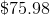, a shirt for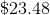, and a pair of pants for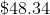. How much did she spend in total? Possible Answers: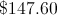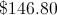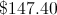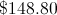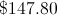Correct answer:Explanation: You add all three values and you get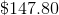. ### Example Question #4 : Problem Solving What is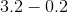? Possible Answers: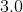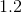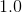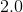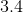Correct answer:Explanation: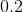removed from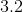results in the whole integer. ### Example Question #5 : Problem Solving Subtract: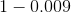Possible Answers: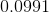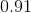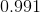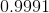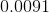Correct answer:Explanation: Rewriteadding the correct number of placeholders and evaluate.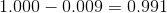### Example Question #6 : Problem Solving Define an operation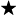on the set of positive integers as follows: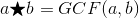If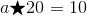, then which of the following couldnot be equal to? Possible Answers:Correct answer:Explanation: If, then the greatest common factor ofand 20 is 10. 80 and 20 both have 20 as a factor - 20 is its own factor, since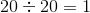, and 20 is a factor of 80, since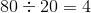. Therefore, 10 is not the greatest common factor of 20 and 80, and 80 is the correct choice. Of the other three factors, it can be seen that the GCF of 20 and each individual number is 10 by looking at each prime factorization: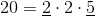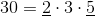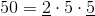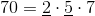In each pairing, the common prime factors are 2 and 5, so the GCF is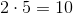. ### Example Question #7 : Problem Solving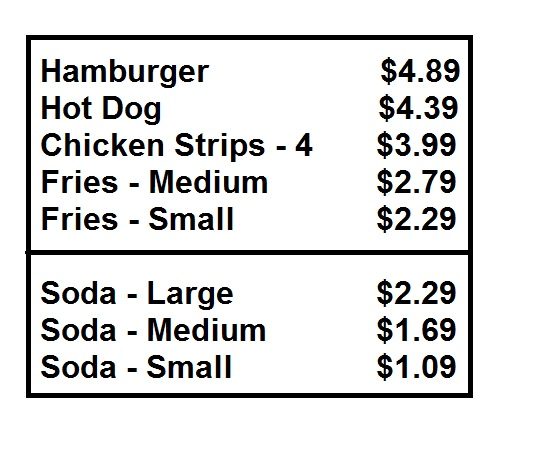The above is a menu at the concession stand at a drive-in movie. Gary wants to order two hamburgers and two small orders of fries. He wants to order two sodas of the same size. If he has just a twenty-dollar bill on hand, what is the largest size soda of which he can order two? Possible Answers: Gary can afford two large sodas Gary can afford two medium sodas, but not two large sodas Gary can afford two small sodas, but not two medium or large sodas Gary cannot afford two sodas Correct answer: Gary can afford two large sodas Explanation: The easiest way to think of this is to note that Gary seems to be ordering for two people, himself and a friend. He has$20, so half of this will be for himself and half for his friend - and half of $20 is$10.

Each hamburger costs $4.89, and each small order of fries costs$2.29. This leaves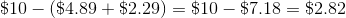to spend on a soda for each person. This will enable to him to buy both himself and his friend a large soda.

### Example Question #8 : Problem SolvingThe above is a menu at the concession stand at a drive-in movie. The stand also offers a Family Chicken Strip Bucket - twenty chicken strips, four medium orders of fries, and four medium sodas for $30. How much more would the items of the Family Chicken Strip Bucket cost if they were purchased separately? (Ignore tax) Possible Answers: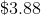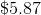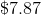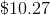Correct answer:Explanation: There are twenty chicken strips in the Family Chicken Strip Bucket, which are the same as in five orders; each order costs$3.99 separately. Each medium order of fries costs $2.79 separately, and each medium soda costs$1.69 separately. The items separately cost a total of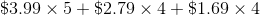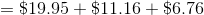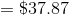Subtract $30; the savings amount to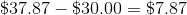### Example Question #9 : Problem SolvingThe above is a menu at the concession stand at a drive-in movie. The stand also offers a special "Meal Deal": a hamburger or a hot dog, a medium order of fries, and a medium soda for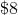Randy orders two Meal Deals - a hamburger Meal Deal for himself and a hot dog Meal Deal for his friend Denise. How much more would he have paid for the items separately? (Ignore tax) Possible Answers: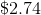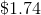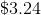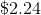Correct answer:Explanation: The two Meal Deals cost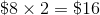. Purchased separately, the hamburger would have cost$4.89; the hot dog, $4.39; each of the two medium orders of fries,$2.79; and each of the two medium sodas, $1.69. The total price of the items, purchased separately, would have been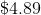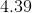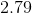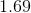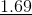The difference between this and the$16 paid for the food is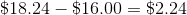### Example Question #10 : Problem SolvingAbove is the menu at the concession stand at a school carnival. Ken wants to purchase a hamburger, a medium order of fries, and medium soda for himself, and a hot dog, a small order of fries, and a small soda for his friend Donna. He hands the clerk a $10 bill and a$5 bill. He has some $1 bills in his pocket; how many of them will he need to hand to the clerk along with the other two bills? (No tax is charged, since this is a school event) Possible Answers: Three Two None One Correct answer: Three Explanation: The items Ken purchases are as follows: Hamburger:$4.89

Medium fries: $2.79 Medium soda:$1.69

Hot dog: $4.39 Small fries:$2.29

Small soda: $1.09 The sum of the prices of these items: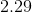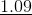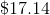Subtract from$20: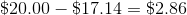Rounding this up - Ken needs to give the clerk three more \$1 bills.

← Previous 1